Maths-
General
Easy

Question

# What is the number that is to be subtracted from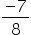𝑡𝑜 𝑔𝑒𝑡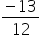Hint:

## The correct answer is: 5/24

### Given rational number =and let the other rational number to be subtracted from=  xOn subtraction, we get the result to beThat is, we have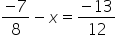On rearranging the equation, we get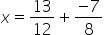On taking LCM of 12 and 8 , we have 24 as the LCM.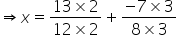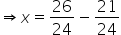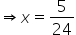#### With Turito Foundation.#### Get an Expert Advice From Turito.